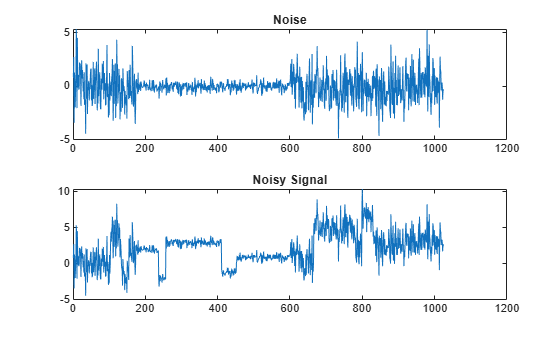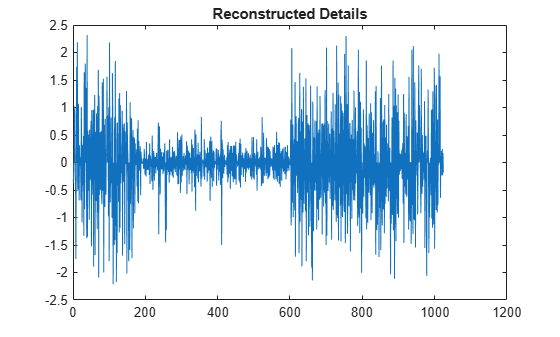# wvarchg

Find variance change points

## Syntax

``[chgpts,kopt,est] = wvarchg(Y)``
``[___] = wvarchg(Y,K)``
``[___] = wvarchg(Y,K,D)``

## Description

example

````[chgpts,kopt,est] = wvarchg(Y)` computes estimated variation change points for the signal `Y` for six change points, where the minimum delay between two change points is 10.```
````[___] = wvarchg(Y,K)` computes estimated variation change points for `j` change points, where `j = 0, 1, 2, …, K`, and the minimum delay between two change points is 10.```
````[___] = wvarchg(Y,K,D)` computes estimated variation change points where the minimum delay between two change points is `D`. `wvarchg(Y,6,10)` is equivalent to `wvarchg(Y)`.`wvarchg(Y,K,10)` is equivalent to `wvarchg(Y,K)`. ```

## Examples

collapse all

For reproducibility, set the random seed to the default value. Load the `blocks` wavelet test signal. Add white noise with two variance change points located at indices 180 and 600. Plot the noise and the noisy signal.

```rng default x = wnoise(1,10); cp1 = 180; cp2 = 600; bb = 1.5*randn(1,length(x)); seg1 = bb(1:cp1); seg2 = bb(cp1+1:cp2)/4; seg3 = bb(cp2+1:end); wn = [seg1 seg2 seg3]; x = x+wn; subplot(2,1,1) plot(wn) title('Noise') subplot(2,1,2) plot(x) title('Noisy Signal')```Use the `db3` wavelet and do a level-1 wavelet decomposition of the signal. Reconstruct the detail coefficients. Replace the top 2% of values with the mean value of the wavelet coefficients to remove most of the signal. Plot the values.

```wname = 'db3'; lev = 1; [c,l] = wavedec(x,lev,wname); det = wrcoef('d',c,l,wname,1); y = sort(abs(det)); v2p100 = y(fix(length(y)*0.98)); ind = find(abs(det)>v2p100); det(ind) = mean(det); figure plot(det) title('Reconstructed Details')```Estimate the variance change points using the wavelet coefficients.

```[pts_Opt,kopt,t_est] = wvarchg(det,5); fprintf('The estimated change points are %d and %d.',pts_Opt)```
```The estimated change points are 181 and 601. ```

## Input Arguments

collapse all

Input signal, specified as a real-valued vector. The input signal `Y` should have zero mean.

Data Types: `double`

Number of change points, specified as an integer. `K` satisfies the inequalities `1 < K ≪ length(Y)`.

Data Types: `double`

Number of change points, specified as an integer. `D` satisfies the inequalities `1 ≤ D ≪ length(Y)`.

Data Types: `double`

## Output Arguments

collapse all

Estimated variance change points, returned as a vector. `chgpts` is the empty vector `[]` when no change points are found.

Proposed number of change points, returned as a nonnegative integer in the interval [0, `k`].

Instants of the variation change points, returned as a real-valued matrix. For `1 ≤ k ≤ K`, `est(k+1,1:k)` contains the `k` instants of the variance change points. If `kopt > 0`, then ```chgpts = est(kopt+1,1:kopt)```, else ```chgpts = []```.

 Lavielle, M. "Detection of multiple changes in a sequence of dependent variables." Stochastic Processes and their Applications. Vol. 83, Number 1, 1999, pp. 79–102.